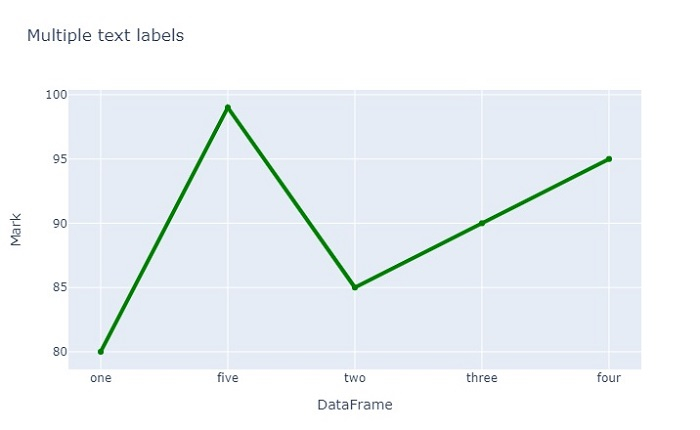# How to add multiple text labels from DataFrame columns in Python Plotly?

Plotly is an open-source plotting library in Python that can generate several different types of charts. Python users can use Plotly to create interactive web-based visualizations.

In this tutorial, we will see how you can use Plotly to add multiple text labels in a chart from DataFrame columns.

• Here, we will use the plotly.graph_objects module to generate figures. It contains a lot of methods to customize charts and render them into HTML format.

• Then, we will use the Scatter() method of this module to generate a scatter plot. The "line" attribute of Scatter() contains a parameter "color" that we will use to specify the required color for the plot.

• To generate the DataFrame, we will use the Pandas module.

Follow the steps given below to add multiple text labels from DataFrame columns.

Step 1

Import the plotly module and alias as py. Similarly, import the pandas module and alias as pd.

import plotly as py
import pandas as pd


Step 2

Import the plotly.graphs_objs module and alias as go.

import plotly.graphs_objs as go


Step 3

Use the Pandas module to create a dataframe.

df = pd.DataFrame({
'Age':[20,22,23,24,21],
'Mark':[80,85,90,95,99],
},


Step 4

Set the index name and sort the values based on the "Age" column,

# Set index name
df.index.name = 'DataFrame'

# Sort the values
df = df.sort_values('Age')


Step 5

Create traces to generate the scatter plot and add a text to show hover on column.

# Create traces to generate scatter plot
# Text to show hover on column
trace = go.Scatter(
x = df.index,
y = df['Mark'],
name = 'Mark',
text = df['Age'],
mode = 'lines+markers',
line = dict(color='green',width = 4)
)


Step 6

Create layout with dict of title for multiple text labels in both X and Y-axis.

layout = dict(
title = 'Multiple text labels',
xaxis = dict(title = 'DataFrame'),
yaxis = dict(title = 'Mark'),
)


Step 7

Generate the figure with the layout values and create an offline plot.

data = [trace]
fig = dict(data=data, layout=layout)
py.offline.plot(fig, filename = 'df.html')


## Example

Here is the complete code to add multiple text labels from DataFrame columns −

import pandas as pd
import plotly as py
import plotly.graph_objs as go

# Crete dataframe
df = pd.DataFrame({
'Age':[20,22,23,24,21],
'Mark':[80,85,90,95,99],
}, index=['one','two','three','four','five'])

# Set index name
df.index.name = 'DataFrame'

# Sort the values
df = df.sort_values('Age')

# Create traces to generate scatter plot
# Text to show hover on column
trace = go.Scatter(
x = df.index,
y = df['Mark'],
name = 'Mark',
text = df['Age'],
mode = 'lines+markers',
line = dict(color='green',width = 4)
)

# Set layout title for X and Y axis
layout = dict(
title = 'Multiple text labels',
xaxis = dict(title = 'DataFrame'),
yaxis = dict(title = 'Mark'),
)

# Create data
data = [trace]

# Set dict of dataset and layout
fig = dict(data=data, layout=layout)

# Generate html file
py.offline.plot(fig, filename = 'df.html')


## Output

It will show the following output on the browser −Updated on: 26-Oct-2022

2K+ Views